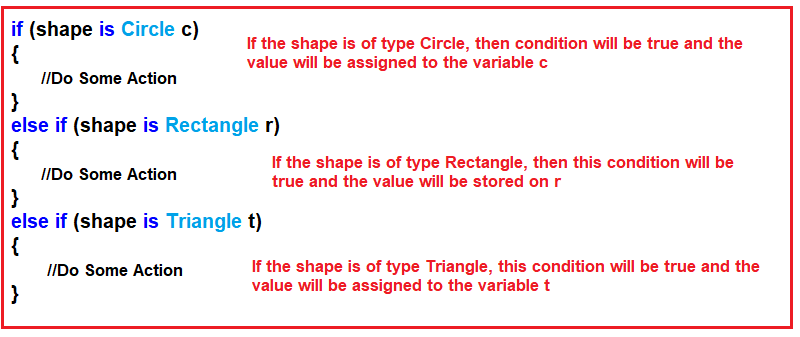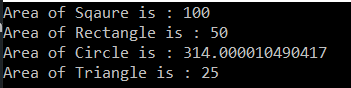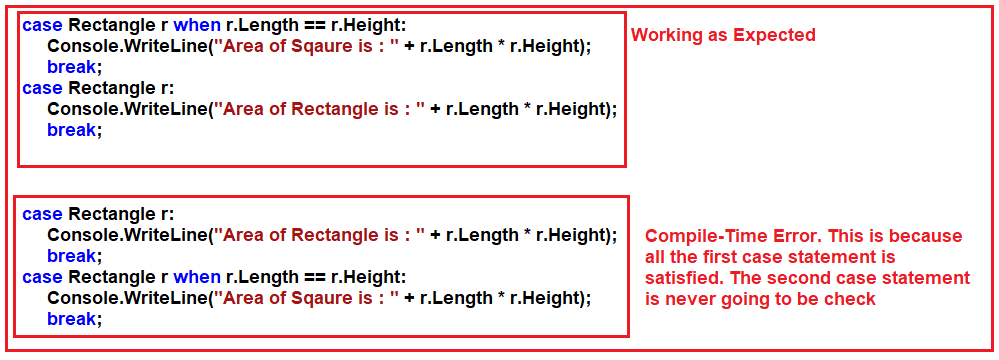# Pattern Matching in C#

## Pattern Matching in C# with Examples

In this article, I am going to discuss Pattern Matching in C# with Examples. Please read our previous article where we discussed the Enhancement of Out Variables in C# 7 with Examples. Pattern Matching is a new feature that was introduced in C# 7.0. As part of this article, we are going to discuss the following pointers.

1. What is Pattern Matching in C#?
2. How to Implement Pattern Matching Before and After C# 7?
3. Pattern Matching Using is Expression.
4. How to Implement Pattern Matching using a switch statement?
5. Understanding the use of  When clauses in the case statement in C#
##### What is Pattern Matching in C#?

Pattern Matching is a mechanism that tests a value i.e. whether the value has a specific shape or not or whether the value is of a specific type or not. If the value is in a specific shape or type then it will extract the data from the value. If this is not clear at the moment, then don’t worry we will understand this with multiple examples.

##### How to implement Pattern Matching in C#?

To implement Pattern Matching in C#, we are provided with two language constructs such as:

1. Pattern Matching using “is” expression
2. The Pattern Matching using “case” statements

In the upcoming versions of C#, we may expect more Pattern Matching expressions. Pattern Matching is useful in many ways however C# 7.0 currently supports the following.

1. It can be used with any data type including the custom data types whereas if/else can only be used with primitive types.
2. Pattern matching has the ability to extract the data from the expression.
##### Pattern Matching in C# with “is” expression:

The “is” operator is available from the first version of C# and it is used to check whether an object is compatible with a specific type or not. For example, if a specific interface is implemented, or if the type of the object derives from a base class or not. The result of this operator is true or false. If this is not clear at the moment then don’t worry, we will try to understand this with some examples.

##### Example to Understand Pattern Matching in C# with “is” Expression

Let us understand how to implement Pattern Matching in C# using the “is” expression with an example. First, create one class with the name Shape, and then create three derived classes with the names Circle, Rectangle, and Tringle as shown below. The following code is very straightforward. Here, we have created one base class i.e. Shape, and three derived classes i.e. Rectangle, Circle, and Triangle, and all these classes are derived from the base Shape class. Further, if you notice, we have created some properties within each derived class and initialized the properties using the class constructor.

```using System;
namespace PatternMatchingDemo
{
//Base Class
public class Shape
{
public const float PI = 3.14f;
}

//Derived Class 1
public class Circle : Shape
{
public double Radius { get; }
{
}
}

//Derived Class 2
public class Rectangle : Shape
{
public double Length { get; }
public double Height { get; }
public Rectangle(double length, double height)
{
Length = length;
Height = height;
}
}

//Derived Class 3
public class Triangle : Shape
{
public double Base { get; }
public double Height { get; }
public Triangle(double @base, double height)
{
Base = @base;
Height = height;
}
}
}
```
##### Pattern Matching Before C# 7:

Let us first understand our requirements. We need to create a method with one parameter of type Shape. The reason is, the Shape class is the base class and it can hold the object reference of any of its child classes like Rectangle, Triangle, and Circle. Please modify the Program class as shown below. Please have a look at the DisplayArea() method. Here in the DisplayArea() method, we are testing each type in a series of “if” and “is” statements, and then we are explicitly casting the type to a specific type and then doing some action.

```using System;
namespace PatternMatchingDemo
{
class Program
{
static void Main()
{
Circle circle = new Circle(10);
DisplayArea(circle);
Rectangle rectangle = new Rectangle(10, 5);
DisplayArea(rectangle);
Triangle triangle = new Triangle(10, 5);
DisplayArea(triangle);
}
public static void DisplayArea(Shape shape)
{
//Checking the actual type of the shape object
if (shape is Circle)
{
//then typecast to actual Circle type
Circle c = (Circle)shape;
Console.WriteLine("Area of Circle is : " + c.Radius * c.Radius * Shape.PI);
}
else if (shape is Rectangle)
{
//then typecast to actual Rectangle type
Rectangle r = (Rectangle)shape;
Console.WriteLine("Area of Rectangle is : " + r.Length * r.Height);
}
else if (shape is Triangle)
{
//then typecast to actual Triangle type
Triangle t = (Triangle)shape;
Console.WriteLine("Area of Triangle is : " + 0.5 * t.Base * t.Height);
}
else
{
throw new ArgumentException(message: "Invalid Shape", paramName: nameof(shape));
}
}
}
}```

Now, let us understand how to use the new Pattern Matching Mechanism which was introduced in C# 7.0.

##### Pattern Matching Using “is” Expression in C# 7:

We can simplify the previous example by using the “is” expression pattern which will check and assign the value to a variable. So, in order to do this, please modify the Program class as shown below. The following code is self-explained, so please go through the comment lines for a better understanding. As you can see in the below code, within the DisplayArea method, type casting to the actual type is eliminated with new Pattern Matching.

```using System;
namespace PatternMatchingDemo
{
class Program
{
static void Main()
{
Circle circle = new Circle(10);
DisplayArea(circle);
Rectangle rectangle = new Rectangle(10, 5);
DisplayArea(rectangle);
Triangle triangle = new Triangle(10, 5);
DisplayArea(triangle);
}
public static void DisplayArea(Shape shape)
{
//If variable shape holds the object of type Circle,
//then it will store that object in variable c
if (shape is Circle c)
{
Console.WriteLine("Area of Circle is : " + c.Radius * c.Radius * Shape.PI);
}

//If variable shape holds the object of type Rectangle,
//then it will store that object in variable r
else if (shape is Rectangle r)
{
Console.WriteLine("Area of Rectangle is : " + r.Length * r.Height);
}

//If variable shape holds the object of type Triangle,
//then it will store that object in variable t
else if (shape is Triangle t)
{
Console.WriteLine("Area of Triangle is : " + 0.5 * t.Base * t.Height);
}
else
{
throw new ArgumentException(message: "Invalid Shape", paramName: nameof(shape));
}
}
}
}```

In the above example, we are using the “is” expressions which will test the variable type and if it matches the type then it assigns that value to the variable. For better understanding please have a look at the following image.##### Pattern Matching using the Switch Statement in C#:

The traditional switch statement in C# is also a Pattern Matching Expression. Let us see how to use the switch statement to implement the previous example. Modify the Program class as shown below to implement Pattern Matching using a Switch Statement in C#. Please have a look at the DisplayArea method.

```using System;
namespace PatternMatchingDemo
{
class Program
{
static void Main()
{
Circle circle = new Circle(10);
DisplayArea(circle);
Rectangle rectangle = new Rectangle(10, 5);
DisplayArea(rectangle);
Triangle triangle = new Triangle(10, 5);
DisplayArea(triangle);
}

public static void DisplayArea(Shape shape)
{
switch (shape)
{
case Circle c:
Console.WriteLine("Area of Circle is : " + c.Radius * c.Radius * Shape.PI);
break;
case Rectangle r:
Console.WriteLine("Area of Rectangle is : " + r.Length * r.Height);
break;
case Triangle t:
Console.WriteLine("Area of Triangle is : " + 0.5 * t.Base * t.Height);
break;
default:
throw new ArgumentException(message: "Invalid Shape", paramName: nameof(shape));
case null:
throw new ArgumentNullException(nameof(shape));
}
}
}
}```
##### Points to Remember while Working with Case Statement in C#:

You need to remember the following points while working with the newly extended switch statement for Pattern Matching in C#.

1. The default clause is always evaluated last: In our example, the null case statement comes at the last but it will be checked before the default case statement is checked. The reason for this is for the compatibility with the existing switch statements. So it is always advisable and a good programming practice to put the default statement at the end.
2. The order of case clauses is now mattered: Just like the catch clauses in the try block, the first one that matches the case statement gets picked. So as a developer, it is important to write the case statement in the proper order.
##### Case Expressions using When Clauses in C#:

Let us understand the use of case Expression using the when clause in C# with an example. In our example, when the length and height both are the same for the rectangle then we need to treat it as a Square and display the message accordingly. We can specify this condition using the when clause. So, modify the Main method and DisplayArea method of the Program class as shown below.

```using System;
namespace PatternMatchingDemo
{
class Program
{
static void Main()
{
Rectangle square = new Rectangle(10, 10);
DisplayArea(square);
Rectangle rectangle = new Rectangle(10, 5);
DisplayArea(rectangle);
Circle circle = new Circle(10);
DisplayArea(circle);
Triangle triangle = new Triangle(10, 5);
DisplayArea(triangle);
}
public static void DisplayArea(Shape shape)
{
switch (shape)
{
case Rectangle r when r.Length == r.Height:
Console.WriteLine("Area of Sqaure is : " + r.Length * r.Height);
break;
case Rectangle r:
Console.WriteLine("Area of Rectangle is : " + r.Length * r.Height);
break;
case Circle c:
Console.WriteLine("Area of Circle is : " + c.Radius * c.Radius * Shape.PI);
break;
case Triangle t:
Console.WriteLine("Area of Triangle is : " + 0.5 * t.Base * t.Height);
break;
default:
throw new ArgumentException(message: "Invalid Shape", paramName: nameof(shape));
}
}
}
}
```

Now, run the application and you should the output as shown below.The most important point that you need to remember is, you need to place the case statement with the when clause first then the normal case statement for the same type. Please have a look at the following diagram for a better understanding.In the next article, I am going to discuss the Digit Separators in C# 7 with Examples. Here, in this article, I try to explain the Pattern Matching in C# using “is” and “case” expressions with examples. I hope you enjoy this Pattern Matching using “is” and “case” expressions articles.

### 4 thoughts on “Pattern Matching in C#”

1.public double Radius { get; }
{
}

Note : if u have only “getter” than how can you set the value in Radius Properties

1.yes i have the same question for that

2.through the constructor set a value…

3.Alok Kumar Sahoo

when you will create the object of circle class , that time pass radius value through constructor.